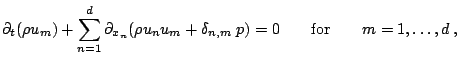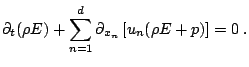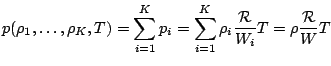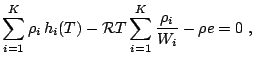Jump:

Start of topic | Skip to actions

Numerical Simulations of Gaseous Detonations

Multi-component Euler Equations

The governing equations of gaseous detonations are the multi-component Euler equations with chemical reactive source terms. These equations can be written in conservation form with K inhomogeneous continuity equationsd momentum equationsand an energy equationAccording to Dalton's law, the total pressure p is the sum of the partial pressures pi, i.e.withFor detailed chemical reaction, all species are usually assumed to be thermally perfect gases with a caloric equationThis model requires the computation of the temperature T from the implicit equationwhenever the pressure has to be evaluated.

Subsections

-- RalfDeiterding - 14 Dec 2004

You are here: Amroc > ClawpackHome > NumSimGasDet > MultiEulerEquations

to top

Copyright © 1997-2019 California Institute of Technology.